Classical Mechanics

# Mechanics Warmups: Level 5 Challenges

Between doing physics problems on Brilliant, some people like to unicycle. A unicyclist is cycling up a hill angled $15^\circ$ with respect to the horizontal. The center of mass of the cyclist is directly over the axle of the wheel and the cyclist/unicycle system have a combined mass of $\SI{100}{\kilo\gram}.$ The radius of the wheel is $\SI{0.5}{\meter}$ and the coefficient of static friction between the wheel and the asphalt is $1.$

What is the magnitude of the torque (in $\si{\newton\meter}$) that the cyclist needs to exert on the pedals in order to cycle up the hill at a constant speed?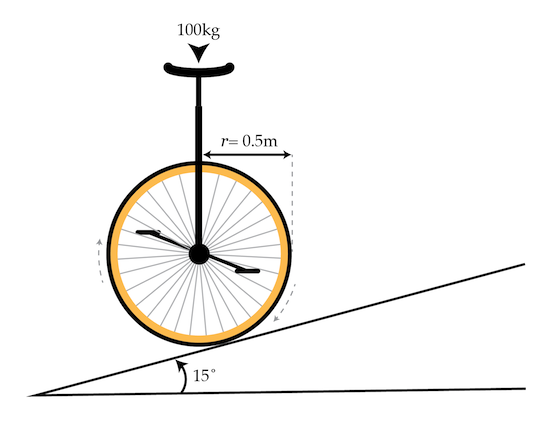Details and assumptions

• The unicycle does not slip against the hill.
• You may take the acceleration of gravity to be $-\SI[per-mode=symbol]{9.8}{\meter\per\second\squared}.$
• You may neglect air resistance.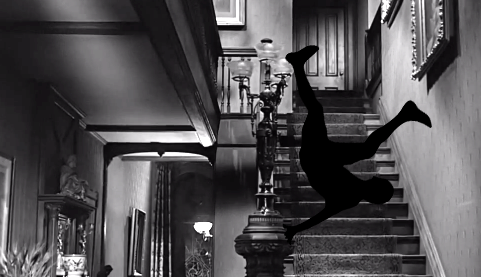A solid homogeneous cylinder of mass $M=1 ~\mbox{kg}$ and radius $R=2~\mbox{m}$ rolls down the stairs as shown in the figure below. The height and the width of the steps are both $h=20~\mbox{cm}$. Assume that the collisions between the cylinder and the steps are inelastic and that the cylinder always remains in contact with the stairs. What will be the terminal velocity (on average) in m/s of the cylinder's center of mass?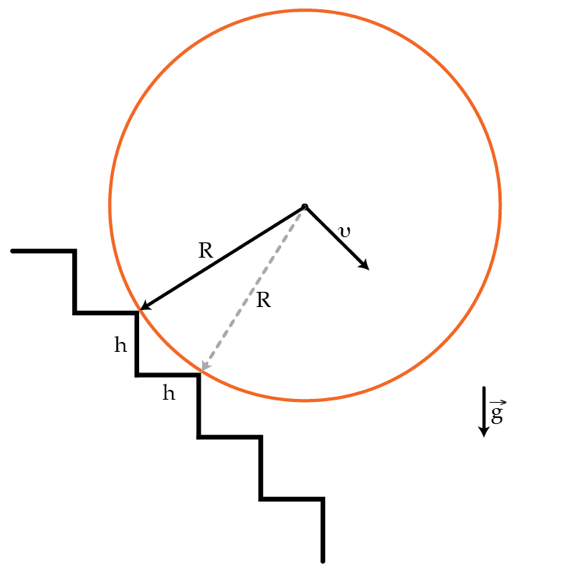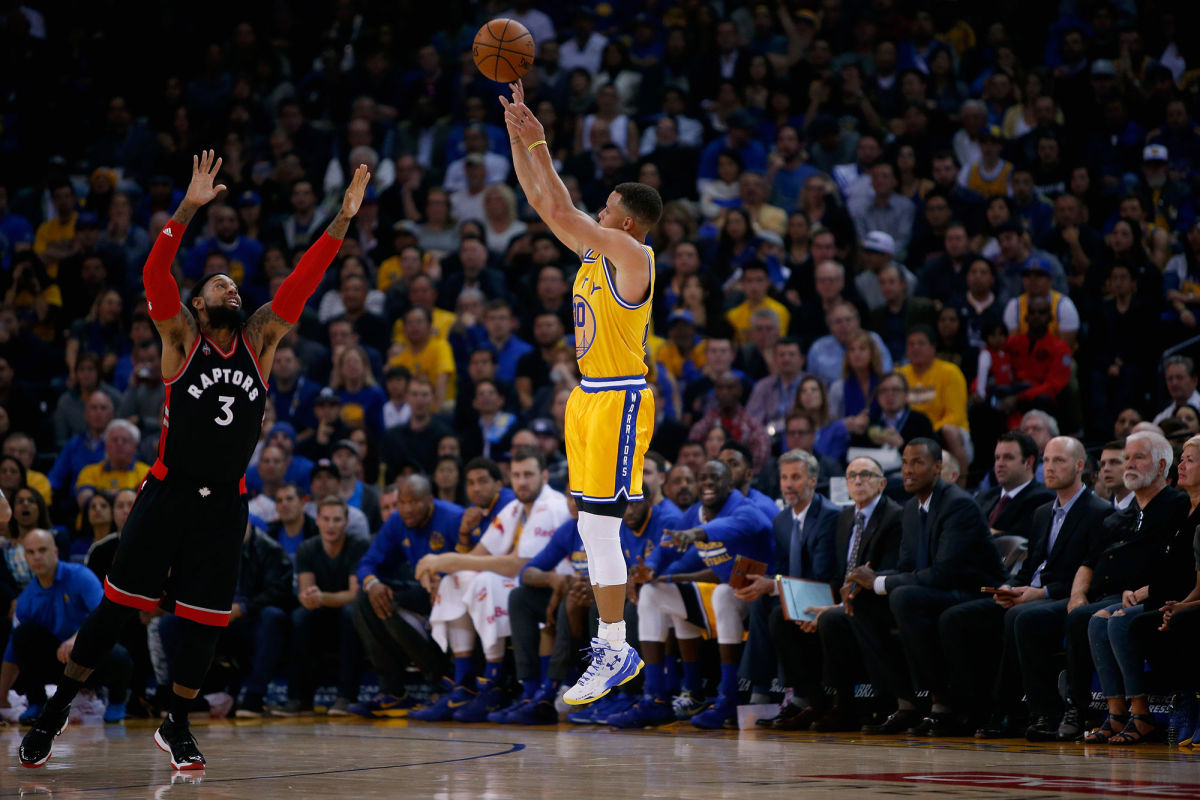Suppose you're taking a three point shot at a basket that's $11 \text{ m}$ down the court, and $1.15 \text{ m}$ above your head. You can take the shot at any angle you like, however, you're not perfect and you're likely to imperfectly set your release angle.

What release angle $\theta$ (in degrees) should you aim for in order to maximize your chance of hitting the three pointer? (In other words, what release angle provides you with the biggest margin of error?)

Details and Assumptions:

• Neglect wind resistance.
• Assume that you aim for the center of the basket.

Phase transitions, like from liquid to solid, are familiar in everyday experience. More exotic phase transitions, such as the change of a conductor to a superconductor, are characterized by a "correlation length." The correlation length is the length over which properties of the system look the same.

As a simple example, consider a line of alternating charges ($+q$ and $-q$), connected by small nonconducting rods to a long vertical rod, as in the figure below. In cylindrical coordinates the charges are at $r=R, z=\ldots -2,-1,0,1,2 \ldots$ and can only rotate around the long rod, i.e. they can only move in $\theta$. At absolute zero, none of the charges can ever have any kinetic energy. Therefore they must all be in their lowest energy state, with all the small rods aligned. Hence for absolute zero the correlation length is infinity, in that if I measure the angle $\theta$ of one rod, I know the angle $\theta$ for a rod an infinite distance away.

If we increase the temperature, then the rods will start to wiggle back and forth. At very high temperatures, the rods are spinning around like mad, and so measuring the angle $\theta$ for one rod tells us nothing about the rod right next to it. In this case the correlation length is zero. The phase transition in this situation is from an uncorrelated or disordered system at non-zero temperature, to a completely ordered system at absolute zero.

Correlation lengths generically diverge with temperature as $L\propto |T-T_c|^{-\nu}$, where $T_c$ is a critical temperature (in our case absolute zero for simplicity), $T$ is near $T_c$ and $\nu$ is a positive number. If we define $L$ for our case as the minimum number of rods between two rods that are oppositely aligned, what is $\nu$ for very low temperatures?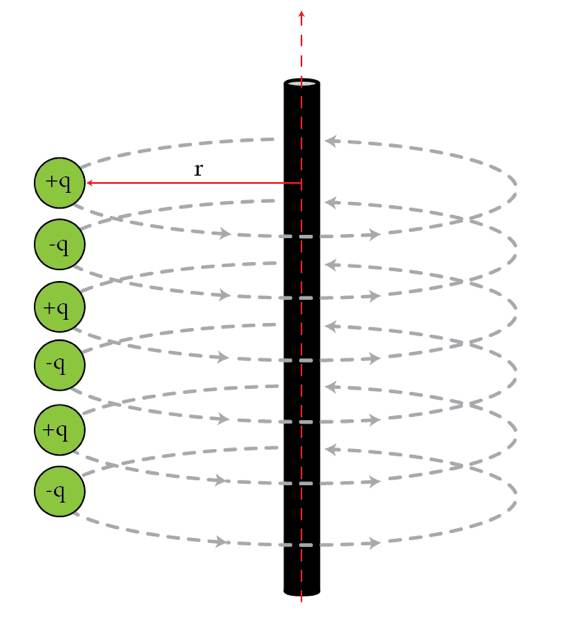This problem is in memoriam of Kenneth Wilson, who developed much of what we know about phase transitions and passed away two weeks ago.

Details and assumptions

• For simplicity, only consider nearest neighbor interactions between the charges.
• Ignore gravity.

A toilet paper roll of mass $m=150~\mbox{g}$ with inner radius $r=1.5~\mbox{cm}$ and outer radius $R=5~\mbox{cm}$ is put on a small cylindrical bar with coefficient of friction $k=2$. If we pull the paper straight down very slowly and increase the force very gradually, the inner part of the roll will first move without slipping on the bar. Then at some critical force $F_c$, it will start to slip. Assume that the pulled out piece of paper is always vertical. Find $F_c$ in Newtons.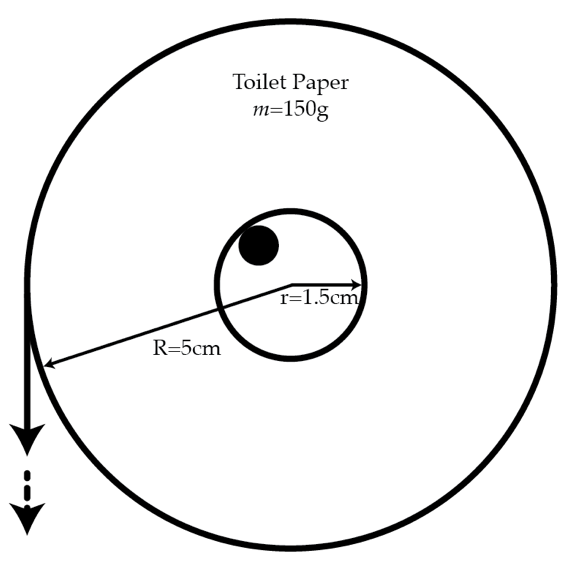Details and assumptions

• The gravitational acceleration is $g=-9.8~\mbox{m/s}^2$
×